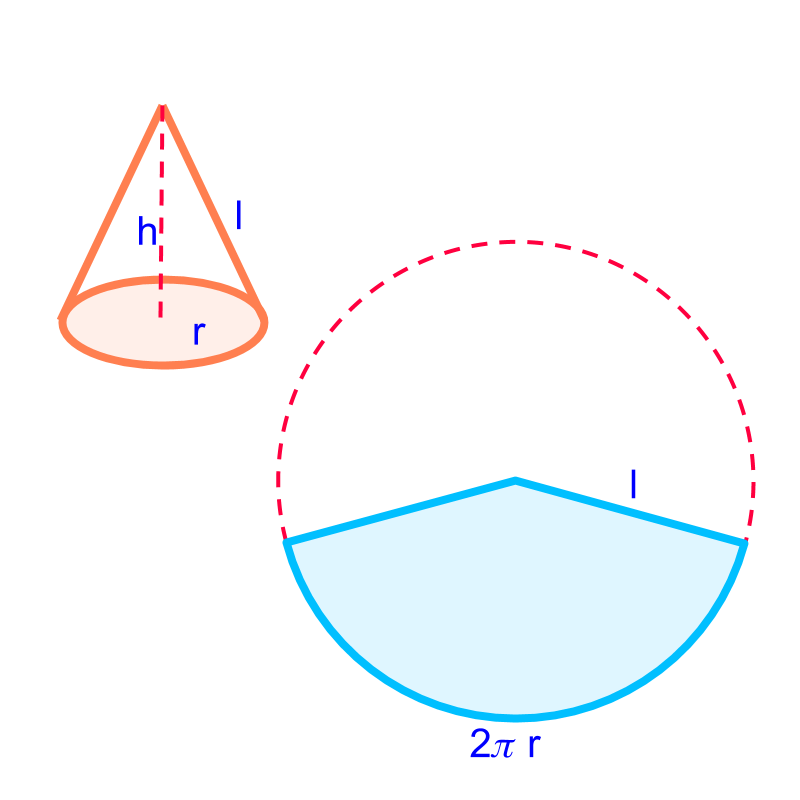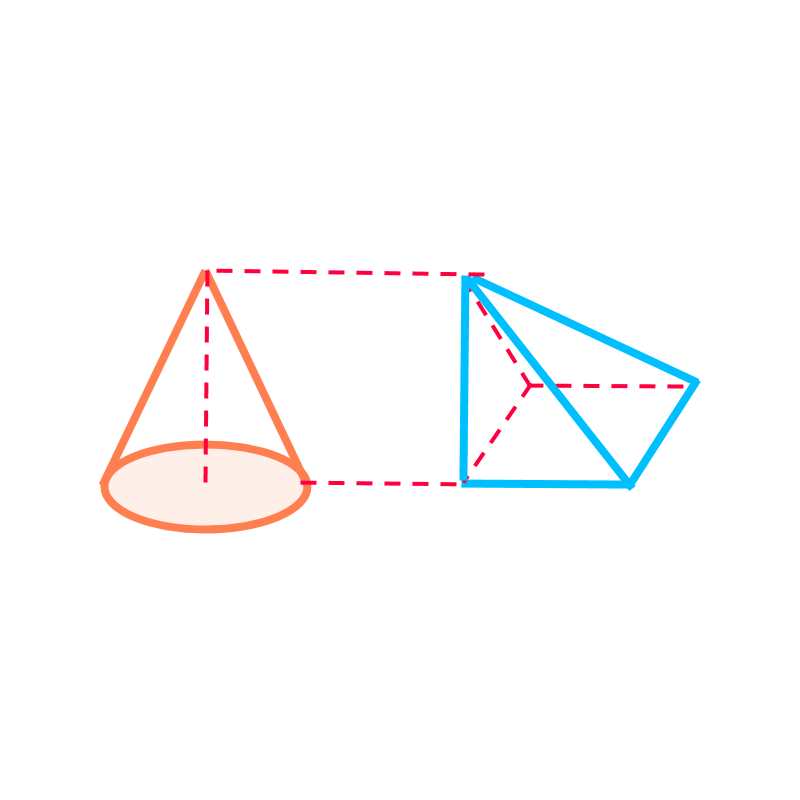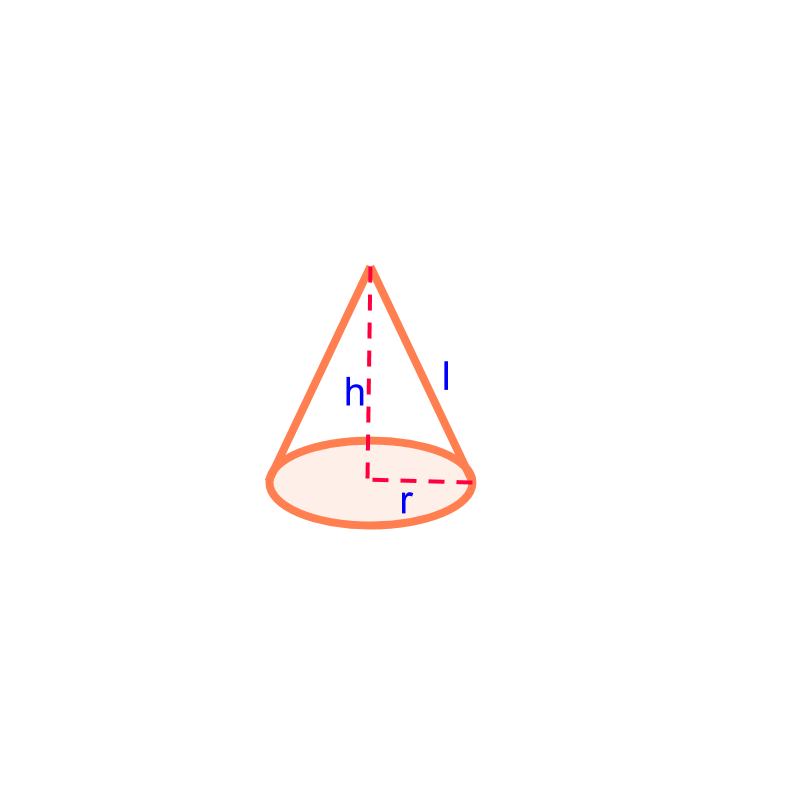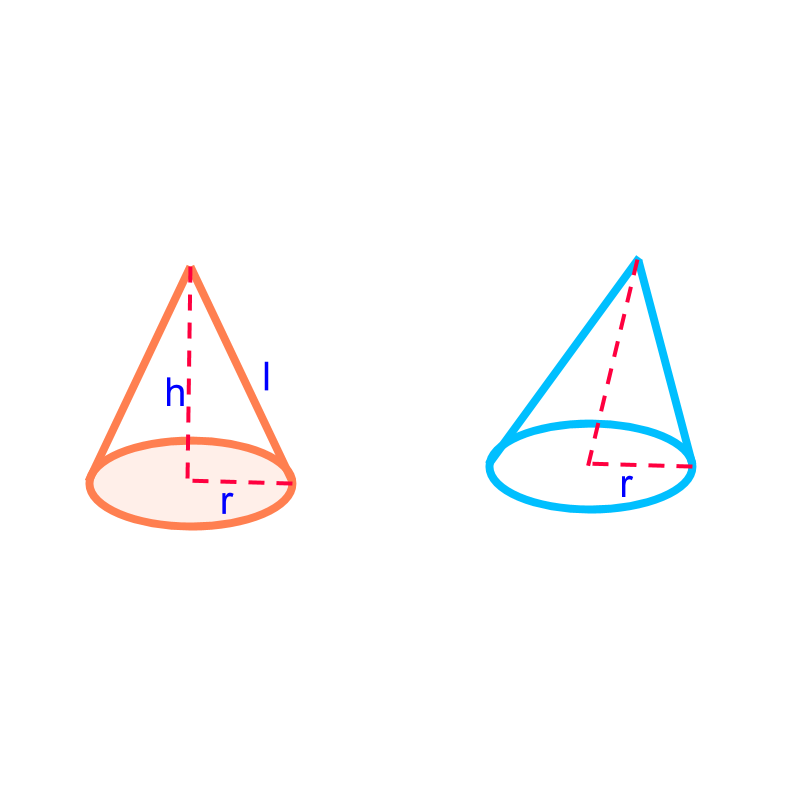maths > mensuration-high

Cone: Surface Area and Volume

what you'll learn...

Overview

Surface Area and Volume of cone:Total surface area of a cone
$=$$=$ area of the base-surface$+$$+$ sum of the area of the side-faces

$=2\pi r\left(r+l\right)$$= 2 \pi r \left(r + l\right)$Volume of a cone
$=\frac{1}{3}×\phantom{\rule{1ex}{0ex}}\text{area of the base-face}\phantom{\rule{1ex}{0ex}}×\phantom{\rule{1ex}{0ex}}\text{height}$$= \frac{1}{3} \times \textrm{a r e a o f t h e b a s e - f a c e} \times \textrm{h e i g h t}$

$=\frac{1}{3}\pi {r}^{2}h$$= \frac{1}{3} \pi {r}^{2} h$

coneThe solid shape shown in the figure is "a cone". Cone is a 3D solid shape with a circular-base at the bottom with a curved surface converging to a single point on the top.We consider only right-circular-cones with its axis at right angle to the base-surface. Other cones are

(1) elliptical-cones with an ellipse at the base-surface

(2) oblique-cones with the angle, between its axis and the base, not a right-angle.

A right-circular-cone is shown in orange. An oblique-cone is shown in blue.

surface areaThe surface area of the cone of radius $r$$r$ and height $h$$h$ is

$\phantom{\rule{1ex}{0ex}}\text{area of base}\phantom{\rule{1ex}{0ex}}$$\textrm{a r e a o f b a s e}$ $+\phantom{\rule{1ex}{0ex}}\text{area of sector}$$+ \textrm{a r e a o f \sec \to r}$

$\phantom{\rule{1ex}{0ex}}\text{area of base}\phantom{\rule{1ex}{0ex}}$$\textrm{a r e a o f b a s e}$ $+\pi \text{radius}×\left(slantheight\right)$$+ \pi \textrm{r a \mathrm{di} u s} \times \left(s l a n t h e i g h t\right)$

$\pi r\left(r+s\right)$$\pi r \left(r + s\right)$

Note: A cone consists of

circular base

a sector from a circle with radius of slant-height $s$$s$ and arc length $2\pi r$$2 \pi r$.

$s$$s$ is the slant height computed as $\sqrt{{r}^{2}/4+{h}^{2}}$$\sqrt{{r}^{2} / 4 + {h}^{2}}$.A cone of height $h$$h$ is shown in orange. The curved lateral surface is unraveled to a sector as shown in blue. The radius of the sector is the slant-height of the cone $s$$s$. And the arc-length of the sector is the perimeter of the base circle of the cone.

Total surface area of the cone

$=$$=$ area of the base-surface $+$$+$ the area of the curved surface

$=\pi {r}^{2}+areaofarc$$= \pi {r}^{2} + a r e a o f a r c$

$=\pi {r}^{2}+\frac{2\pi r}{2\pi s}×\pi {s}^{2}$$= \pi {r}^{2} + \frac{2 \pi r}{2 \pi s} \times \pi {s}^{2}$

$=\pi {r}^{2}+\pi rs$$= \pi {r}^{2} + \pi r s$

`= pi r (r + s)

volume

The volume of the cone of radius $r$$r$ and height $h$$h$ is

volume of the modified-square-pyramid

$\frac{1}{3}×\phantom{\rule{1ex}{0ex}}\text{area of base-surface}\phantom{\rule{1ex}{0ex}}$$\frac{1}{3} \times \textrm{a r e a o f b a s e - s u r f a c e}$ $×\phantom{\rule{1ex}{0ex}}\text{height}$$\times \textrm{h e i g h t}$

$\frac{1}{3}\pi {r}^{2}h$$\frac{1}{3} \pi {r}^{2} h$The cone is shown in orange. As per the Cavalieri's principle in 3D, the cone is equivalently represented by the oblique-pyramid shown in blue.

The modified-pyramid has

•  the area at the base surface equal to the area of base-surface of the cone

•  the height equal to the height of the cone.

•  the cross-sectional area along the vertical axis equal to that of the cone

Volume of the cone

$=$$=$ volume of the pyramid with identical cross-sectional area along the vertical axis

$=\frac{1}{3}×\phantom{\rule{1ex}{0ex}}\text{area of the base}\phantom{\rule{1ex}{0ex}}×\phantom{\rule{1ex}{0ex}}\text{height}$$= \frac{1}{3} \times \textrm{a r e a o f t h e b a s e} \times \textrm{h e i g h t}$

$=\frac{1}{3}×\pi {r}^{2}×h$$= \frac{1}{3} \times \pi {r}^{2} \times h$

$=\frac{1}{3}\pi {r}^{2}h$$= \frac{1}{3} \pi {r}^{2} h$

What is the volume of a cone of height $7$$7$ cm and radius $3$$3$ cm?
The answer is "$66c{m}^{3}$$66 c {m}^{3}$"

summary

Surface Area and Volume of cone:Total surface area of a cone
$=$$=$ area of the base-surface$+$$+$ sum of the area of the side-faces

$=2\pi r\left(r+l\right)$$= 2 \pi r \left(r + l\right)$Volume of a cone
$=\frac{1}{3}×\phantom{\rule{1ex}{0ex}}\text{area of the base-face}\phantom{\rule{1ex}{0ex}}×\phantom{\rule{1ex}{0ex}}\text{height}$$= \frac{1}{3} \times \textrm{a r e a o f t h e b a s e - f a c e} \times \textrm{h e i g h t}$

$=\frac{1}{3}\pi {r}^{2}h$$= \frac{1}{3} \pi {r}^{2} h$

Outline